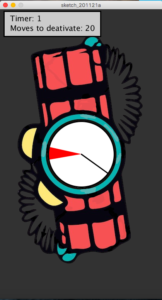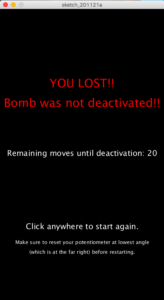# Don’t let it explode – Week 10

#### introduction

For this week’s assignment, the first thing I started thinking about was which elements to use from Arduino. I wanted Arduino to offer some sort of functionality that Processing couldn’t.
So, I decided to use a potentiometer that would allow you to rotate a line within a circle, by converting the potentiometer values into angles.
Yes, this can be done with just Processing, but I think that physically twisting a button and having the line rotate as you twist, is something you can’t replicate with the keyboard or mouse.

#### idea

The point of the game is to try and deactivate a bomb before it explodes.
A red arc shows up randomly on the screen of the bomb and you have to move the potentiometer to get the line inside that arc before a specific amount of time passes.
When you first run the game, you get around 5 seconds to deactivate the first arc. This is because it was taking some time at first to load everything. But after that, you have 2 seconds for each arc.
You would win/deactivate the bomb if you are able to deactivate 20 arcs. And, you would lose if 2 seconds go by before you are able to reach one of the arcs.
You can restart the game by clicking the mouse anywhere on the screen.Here, you can see how the potentiometer controls the line:

#### Circuit

The wiring is really basic. All I used was a potentiometer.

#### code

This the Arduino Code:

```//get value of potentiometer at where you want degree 180 to be
float at180=862;
//get value of potentiometer at where you want degree 0 to be
float at0=144;

void setup() {
// put your setup code here, to run once:
Serial.begin(9600);
Serial.println('0'); //sending an ASCII version
//println sends a string of ascii values
}

void loop() {

if(Serial.available()>0)
{
float toAngle = ((at180-at0)/180);
//convert it to an angle
float angle = (sensor - at0) / toAngle;
delay(1);
//send the angle value to processing
Serial.println(String(angle));
}
}```

And this is the Processing code:

```import processing.serial.*;
Serial myPort;
float pAngle;
PImage bomb;
int x =0;
int y =0;
int r = 170;
float angle, dist;
boolean done, lost, won;
color c;
int score;
int timer, timeleft, timestart,seconds;
int coolingTime;
float lineX, lineY;

void setup()
{
size(420,750);
//connection with arudino
printArray(Serial.list());
String portname=Serial.list();
println(portname);
myPort = new Serial(this,portname,9600);
myPort.clear();
myPort.bufferUntil('\n');
//variable initialization
lost = false;
won = false;
done = false;
angle = random (-8*PI/7,PI/6); //radnom angle
dist = angle + PI/8; //another angle distant from the first one by PI/8 to make up the arc
c = color(255,0,0);
score=0;
timer = timeleft = 5500; // set timer for about 5 seconds the first time
//because it takes some time to load the potentiometer
//but then you get only 2 seconds
timestart = millis();
coolingTime =0; //variable used to pause a bit before switching to another random angle value
}

void draw(){
//display once you lose
if (lost)
{
background(0);
fill(255,0,0);
textSize(30);
textAlign(CENTER);
text("YOU LOST!!", width/2,height/3 - 50);
text("Bomb was not deactivated!!", width/2,height/3);
textSize(20);
fill(255);
text("Remaining moves until deactivation: "+ str(20-score), width/2,height/2);
textSize(20);
text("Click anywhere to start again.", width/2,3*height/4);
textSize(13);
text("Make sure to reset your potentiometer at lowest angle", width/2,4*height/5);
text("(which is at the far right) before restarting.", width/2,5*height/6);
}
//display once you won
else if (won)
{
background(100);
fill(0,255,0);
textSize(50);
textAlign(CENTER);
text("BOMB", width/2,height/3);
text("DEACTIVATED", width/2,height/3 + 60);
fill(255);
textSize(20);
text("Click anywhere to start again.", width/2,3*height/4);
textSize(13);
text("Make sure to reset your potentiometer at lowest angle", width/2,4*height/5);
text("(which is at the far right) before restarting.", width/2,5*height/6);
}
//display during the game
else if (!won && !lost)
{
background(50);
image(bomb,-2,4);
translate(width/2,height/2);
timeleft =  timer - (millis() - timestart);
seconds = (timeleft/1000) % 60;
//println(seconds);
fill (255,190);
rect(-200,-370,250,65);
fill(0);
textSize(20);
textAlign(LEFT);
text("Timer: "+ seconds, -185, -345);
text("Moves to deativate: "+ str(20-score), -185, -320);

//drawing a circle and the arc
strokeWeight(3);
fill(255);
ellipse(0,0,r,r);
fill(c);
arc(x, y, r, r, angle, dist);
//drawing the line controlled by the potentiometer angle value
lineX = cos(pAngle)*(r/2);
lineY = sin(pAngle)*(r/2);
line(0,0,lineX,lineY);
//losing condition : you run out of time before reaching an arc
if (seconds <= 0)
{
lost = true;
}
//winning condition : you manage to reach 20 arcs
if (score == 20)
{
won = true;
}
//if you touch an arc before timer runs out
if ((pAngle>angle)&&(pAngle<dist) && seconds>0){
done = true;
c= color(0,255,0); //change color to green
coolingTime ++; //start incrementing coolingTime
timer = timeleft = 2500; //restart the timer
timestart = millis();
}
//this statement is used so that cooling time will still increment if you touch the arc
//and then move away from it
else if ( !((pAngle>angle)&&(pAngle<dist)) && coolingTime >= 1) {coolingTime++;}
//println(coolingTime);
//once the coolingTime is over
if(coolingTime> 15)
{
score++; //increment score
//get new random arc
angle = random (-8*PI/7,PI/6);
dist = angle + PI/8;
done = false;
c= color(255,0,0); //change color of arc back to red
coolingTime = 0; //reset coolingTime to 0
}
}
}
//restart the game and resee all the needed variables when mouse is clicked at one of the end screens
void mouseClicked()
{
if (lost || won)
{
won = false;
lost = false;
timer = timeleft = 5500;
timestart = millis();
score=0;
coolingTime = 0;
done = false;
angle = random (-8*PI/7,PI/6);
dist = angle + PI/8;
c = color(255,0,0);
}
}

//getting the value from arduino
void serialEvent(Serial myPort){
String s=myPort.readStringUntil('\n'); //to know where it ends
s=trim(s);
if (s!=null){
pAngle= int(s);
}
//println(pAngle);
myPort.write("0"); //send something back to arduino to ask for next value
}```

#### final outcome

If I had more time, I would have liked to add a buzzer, as well as a button on Arduino to restart the game instead of doing that with the mouse. I would have also liked to add a start-screen.
Something else I would like to fix is when generating a random arc, it shouldn’t be where the previous one was.

Anyway, this is the final outcome.

Posted on Categories Fall 2020

## 2 thoughts on “Don’t let it explode – Week 10”

1.Aaron says:

Great job Ons.

1.Ons Taktak says:

Thank you!